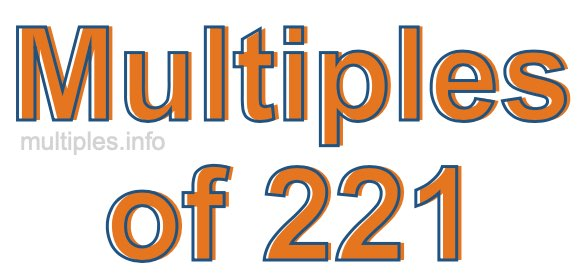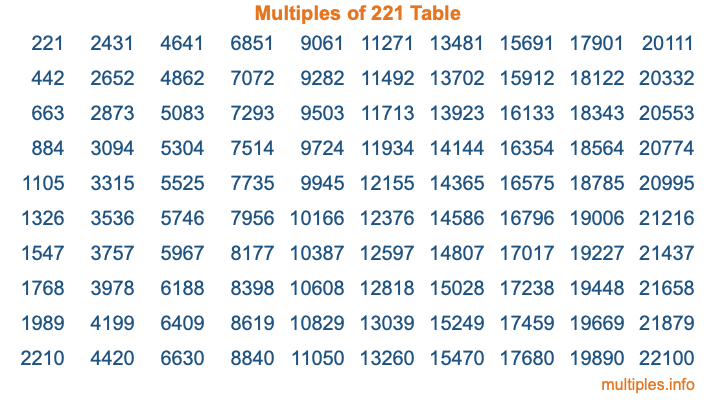Multiples of 221Welcome to the Multiples of 221 page. Here we will first teach you everything you will ever need to know about the multiples of 221, and then give you a study guide summary of everything we taught you to make sure you remember it all. Use this page to look up facts and learn information about the multiples of 221. This page will make you a multiples of two hundred twenty-one expert!

Definition of Multiples of 221
Multiples of 221 are all the numbers that when divided by 221 equal an integer. Each of the multiples of 221 are called a multiple. A multiple of 221 is created by multiplying 221 by an integer.

Therefore, to create a list of multiples of 221, you start with 1 multiplied by 221, then 2 multiplied by 221, then 3 multiplied by 221, and so on for as long as you want. Thus, the list of the first five multiples of 221 is 221, 442, 663, 884, and 1105. To see a larger list of multiples of 221, see the printable image of Multiples of 221 further down on this page. We also have a category where you can choose any nth multiple of 221.

Multiples of 221 Checker
The Multiples of 221 Checker below checks to see if any number of your choice is a multiple of 221. In other words, it checks to see if there is any number (integer) that when multiplied by 221 will equal your number. To do that, we divide your number by 221. If the the quotient is an integer, then your number is a multiple of 221.

Is  a multiple of 221?

Least Common Multiple of 221 and ...
A Least Common Multiple (LCM) is the lowest multiple that two or more numbers have in common. This is also called the smallest common multiple or lowest common multiple and is useful to know when you are adding our subtracting fractions. Enter one or more numbers below (221 is already entered) to find the LCM.

Check out our LCM Calculator if you need more details about the Least Common Multiple or if you need the LCM for different numbers for adding and subtraction fractions.

nth Multiple of 221
As we stated above, 221 is the first multiple of 221, 442 is the second multiple of 221, 663 is the third multiple of 221, and so on. Enter a number below to find the nth multiple of 221.

th multiple of 221

Multiples of 221 vs Factors of 221
221 is a multiple of 221 and a factor of 221, but that is where the similarities end. All postive multiples of 221 are 221 or greater than 221. All positive factors of 221 are 221 or less than 221.

Below is the beginning list of multiples of 221 and the factors of 221 so you can compare:

Multiples of 221: 221, 442, 663, 884, 1105, etc.

Factors of 221: 1, 13, 17, 221

As you can see, the multiples of 221 are all the numbers that you can divide by 221 to get a whole number. The factors of 221, on the other hand, are all the whole numbers that you can multiply by another whole number to get 221.

It's also interesting to note that if a number (x) is a factor of 221, then 221 will also be a multiple of that number (x).

Multiples of 221 vs Divisors of 221
The divisors of 221 are all the integers that 221 can be divided by evenly. Below is a list of the divisors of 221.

Divisors of 221: 1, 13, 17, 221

The interesting thing to note here is that if you take any multiple of 221 and divide it by a divisor of 221, you will see that the quotient is an integer.

Multiples of 221 Table
Below is an image of the first 100 multiples of 221 in a table. The table is in chronological order, column by column. The first column has the first ten multiples of 221, the second column has the next ten multiples of 221, and so on.The Multiples of 221 Table is also referred to as the 221 Times Table or Times Table of 221. You are welcome to print out our table for your studies.

Negative Multiples of 221
Although not often discussed or needed in math, it is worth mentioning that you can make a list of negative multiples of 221 by multiplying 221 by -1, then by -2, then by -3, and so on, to get the following list of negative multiples of 221:

-221, -442, -663, -884, -1105, etc.

Multiples of 221 Summary
Below is a summary of important Multiples of 221 facts that we have discussed on this page. To retain the knowledge on this page, we recommend that you read through the summary and explain to yourself or a study partner why they hold true.

There are an infinite number of multiples of 221.

A multiple of 221 divided by 221 will equal a whole number.

221 divided by a factor of 221 equals a divisor of 221.

The nth multiple of 221 is n times 221.

The largest factor of 221 is equal to the first positive multiple of 221.

221 is a multiple of every factor of 221.

221 is a multiple of 221.

A multiple of 221 divided by a divisor of 221 equals an integer.

221 divided by a divisor of 221 equals a factor of 221.

Any integer times 221 will equal a multiple of 221.

Multiples of a Number
Here you can get the multiples of another number, all with the same attention to detail as we did for multiples of 221 on this page.

Multiples of
Multiples of 222
Did you find our page about multiples of two hundred twenty-one educational? Do you want more knowledge? Check out the multiples of the next number on our list!